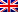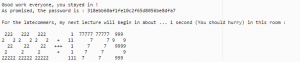#[NDH 2016] [Web 250 – Classroom] Write Up

## Description

We were facing a service that was hiding himself, jumping from port to port

# Question: Follow the course, get the Flag

## Resolution

This one was a challenge, because all we had was an address but no port to connect to :

`"classroom.wargame.ndh"`

So we had to start by finding the service. that can be done quickly with a small port scan of the host :

```cpt=1024; while [ \$cpt -ne 2048 ]; do echo \$cpt; nc classroom.wargame.ndh \$cpt; cpt=\$((\$cpt+1));done

```

Once connected, we immediatly receive a big big bunch of raw datas, that looks like an hexadecimal string.

once we were done receiving datas, and if we stayed connected long enough, we end up receiving this message :So it looks like we missed the begining of the class, so we couldn’t get the whole “lesson”. but a new one is starting … we shall try to connect to it.

This is we the challenge was (i guess) broken.

almost 99% of the time 2001 was the port we were connected on, and that was still the port we were supposed to connect to.

I guess the admin were trying to make the service jump from port to port, so we had to solve the ascii-art math to know where to connect to.

was it easier then … not totally.

because the service was still dropping the connection and restarting on the new port (the same 2001 port), if we were to connect to it to quickly, we would still end up beeing disconnected.

that was not very interresting, so i won’t go into a lots of detail. just know that a sleep(2) was the right timing for us.

even though, before we could realize the challenge was kinda broken, we implemented a way to solve the ascii-art, so here it is :

the idea was to only look at the line wich contain a ‘+’ or a ‘-‘ to solve the math.

here is our dirty but quick and easy way to do so :

```
while 1:
line = sh.recvuntil('\n')
if '+' in line:
print line
res=calculate_plus(line)
PORT=int(res)
sh.close()
sleep(2)
break
elif '-' in line:
print line
res=calculate_moins(line)
PORT=int(res)
sh.close()
sleep(2)
break
else :
print line

#The first '+' is on the second line of the ascii art. so we just have to get the values and deal with how large they are on that line
def calculate_plus(line):
val=''
values=[]
index=0
while index < len(line):
if line[index]=='1':
val+='1'
index+=1
elif line[index]=='2':
val+='2'
index+=4
elif line[index] == '0':
val += '0'
index += 4
elif line[index] == '3':
val += '3'
index += 4
elif line[index] == '4':
val += '4'
index += 1
elif line[index] == '5':
val += '5'
index += 1
elif line[index] == '6':
val += '6'
index += 1
elif line[index] == '7':
val += '7'
index += 0
elif line[index] == '8':
val += '8'
index += 4
elif line[index] == '9':
val += '9'
index += 4
elif line[index] == '+':
values.append(val)
val = ''
index += 1
index+=1
values.append(val)
print "Calculating : %i + %i = %i"%(int(values),int(values),int(values)+int(values))
final=int(values)+int(values)
return final

#The first '-' is on the third line of the ascii art. so we just have to get the values and deal with how large they are on that line too
def calculate_moins(line):
val=''
values=[]
index=0
while index < len(line):
if line[index]=='1':
val+='1'
index+=1
elif line[index]=='2':
val+='2'
index+=1
elif line[index] == '0':
val += '0'
index += 4
elif line[index] == '3':
val += '3'
index += 1
elif line[index] == '4':
val += '4'
index += 2
elif line[index] == '5':
val += '5'
index += 3
elif line[index] == '6':
val += '6'
index += 3
elif line[index] == '7':
val += '7'
index += 0
elif line[index] == '8':
val += '8'
index += 2
elif line[index] == '9':
val += '9'
index += 3
elif line[index] == '-':
values.append(val)
val = ''
index += 3
index+=1
values.append(val)
print "Calculating : %i - %i = %i" % (int(values), int(values), int(values) - int(values))
final=int(values)-int(values)
return final
```

With that we could finally connect to the new classroom with the right timing.

we were welcomed with a message saying someting like : “The lesson is about to start. at the end you will receive the password (reversed)”

then the big bunch of raw datas that we were used to received started to flow.

as usual, we gathered it all, and waited for the end of the classroom to get the password.

with “all” the datas, as hexadecimal value, we could finally get the magic header and identify it as a 7z archive (the header start with 0x37 0x7A wich is 7z).

we just had to recreate a file with a pretty standard :

```
datas = binascii.unhexlify(raw_datas)
f=open('rawfile.7z','wb')
f.write(datas)
f.close()
```

we then just had to insert the password in our favorite 7z unarchiver. (the reversed password, don’t forget)We actually almost cried when we saw this picture, because it was like 2 minutes from the end of the contest and we though “shit, there is still a stega to get the flag !”

but no, there was a second file in the archive

`flag{ndh2k16_a9e316ac765efdee63d51e170ecf057b}`